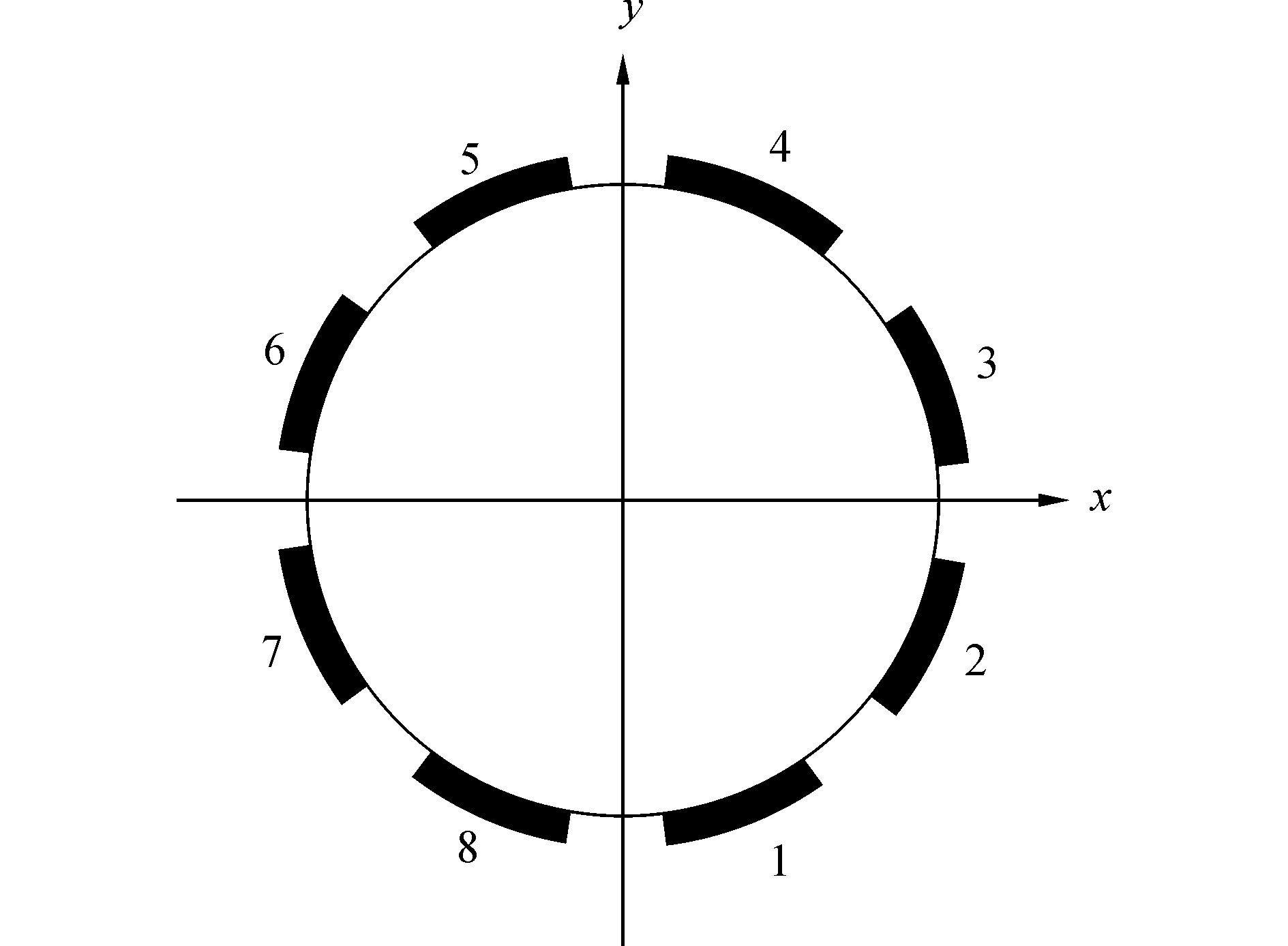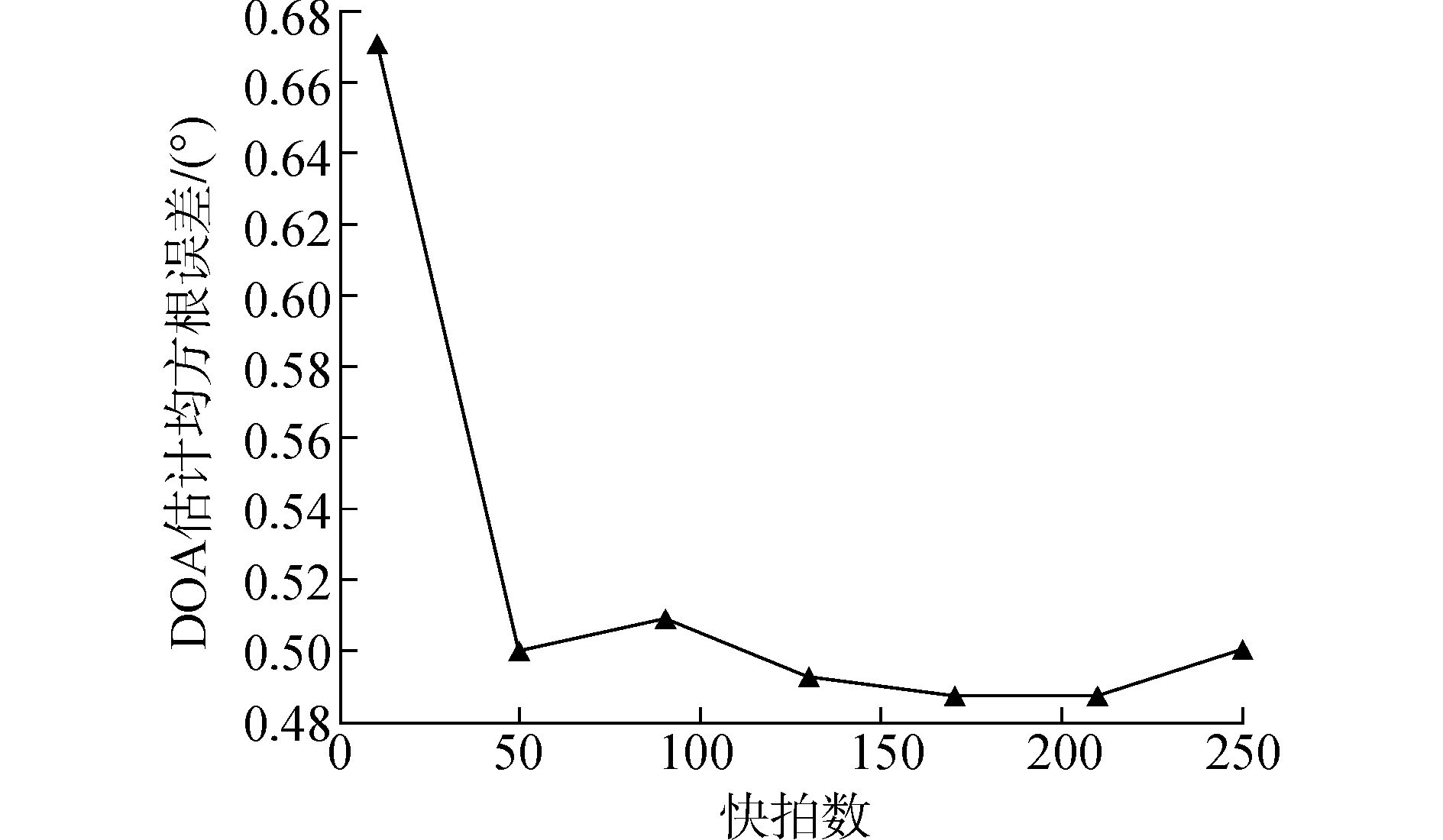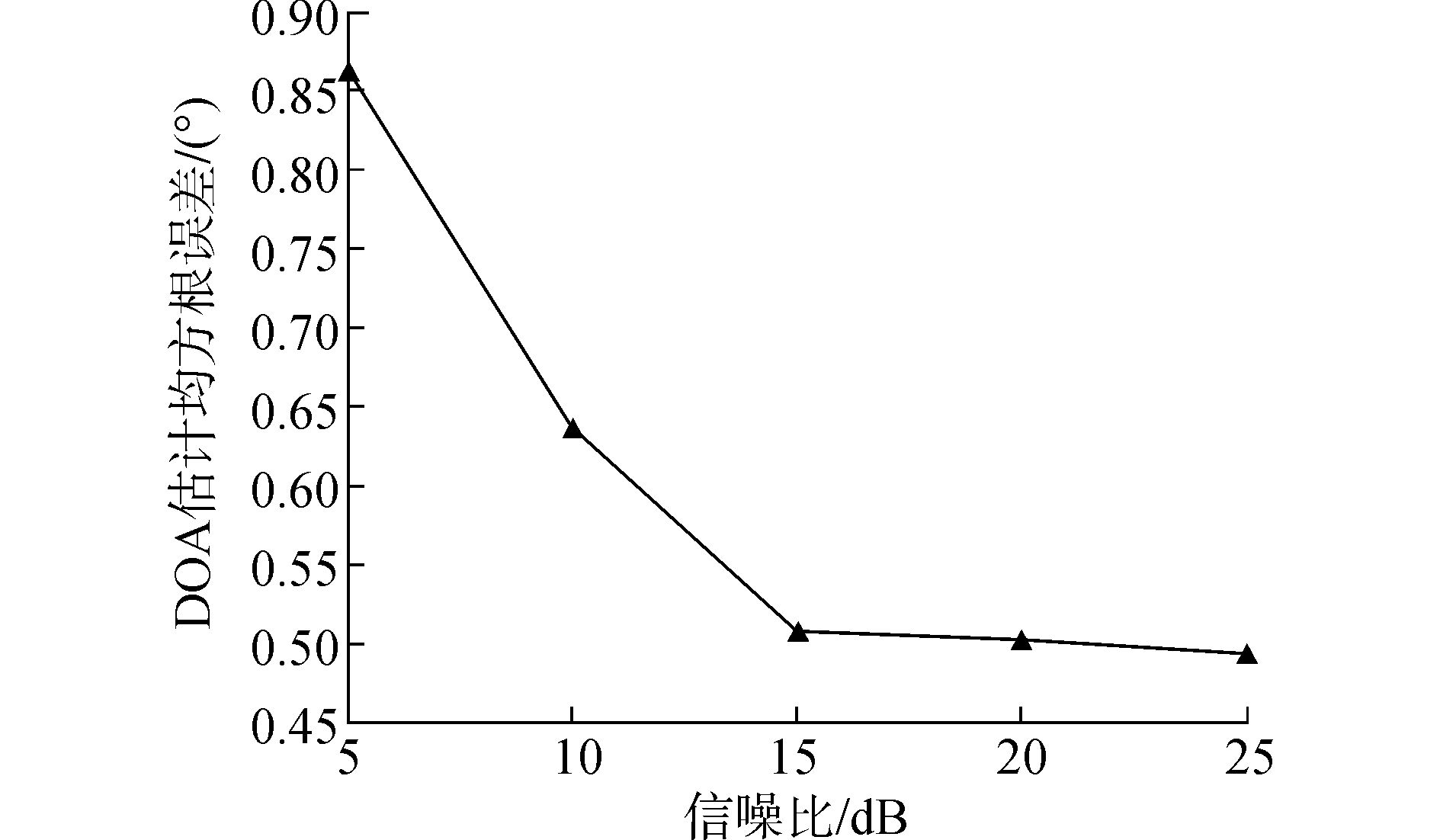﻿ 极化敏感阵列的DOA及极化参数降维估计算法
«上一篇文章快速检索 高级检索

 应用科技2017, Vol. 44Issue (3): 39-42, 90  DOI: 10.11991/yykj.2016050160

### 引用本文ZENG Fuhong, QU Zhiyu, SI Weijian. Dimension-reduction for DOA and polarization estimation based on polarization sensitive array[J]. Applied Science and Technology, 2017, 44(3), 39-42, 90. DOI: 10.11991/yykj.201605016.### 文章历史

Dimension-reduction for DOA and polarization estimation based on polarization sensitive array
ZENG Fuhong, QU Zhiyu, SI Weijian
College of Information and Communication Engineering, Harbin Engineering University, Harbin 150001, China
Abstract: To solve the problem that polarization MUSIC algorithm has large computational complexity, a rank loss MUSIC algorithm suitable for polarization sensitive array was proposed.On the basis of polarization MUSIC algorithm, using the principle of matrix rank loss to reduce the dimensionality of the spectral function to two-dimensional spectral function related to the airspace parameters, the computation significantly was decreased but also guarantees the direction of arrival (DOA) estimation accuracy.After getting the DOA of the incident signal, the polarization parameters can be directly calculated by formula which has low computational complexity.Simulation results show that the proposed algorithm has high estimation precision.The proposed algorithm has better real-time performance compared with that polarization MUSIC algorithm.
Key words: DOA estimation    polarization sensitive array    polarization MUSIC algorithm    rank loss MUSIC algorithm    dimensionality reduction optimization    real-time performance    estimation precision

1 数学模型图 1 八元均匀圆阵示意图

 $\mathit{\boldsymbol{X}}\left( t \right) = \sum\limits_{m = 1}^D {{\mathit{\boldsymbol{a}}_m}{\mathit{\boldsymbol{s}}_m}\left( t \right)} + \mathit{\boldsymbol{N}}\left( t \right) = \mathit{\boldsymbol{AS}}\left( t \right) + \mathit{\boldsymbol{N}}\left( t \right)$ (1)

 ${u_i} = {{\rm{e}}^{^ - {\rm{j}}2{\rm{ \mathsf{ π} }}/\lambda ({\mathit{\boldsymbol{l}}_i}{\mathit{\boldsymbol{r}}^{\rm{T}}})}},{\rm{ }}\left( {i = 1,2, \cdots ,8} \right)$ (2)

 $\mathit{\boldsymbol{a}} = \mathit{\boldsymbol{r}}\left( {\theta ,\varphi } \right)\mathit{\boldsymbol{B}}\mathit{\boldsymbol{\phi}}\left( {\theta ,{\rm{ }}\varphi } \right)\mathit{\boldsymbol{h}}\left( {\gamma ,\eta } \right)$ (3)

 $\mathit{\boldsymbol{\phi}}\left( {\theta ,\varphi } \right) = \left[ {\begin{array}{*{20}{c}} { - {\rm{sin}}\theta } & {{\rm{sin}}\varphi {\rm{cos}}\theta }\\ {{\rm{cos}}\theta } & {{\rm{sin}}\varphi {\rm{sin}}\theta }\\ 0 & { - {\rm{cos}}\varphi }\\ {{\rm{sin}}\varphi {\rm{cos}}\theta } & {{\rm{sin}}\theta }\\ {{\rm{sin}}\varphi {\rm{cos}}\theta } & { - {\rm{cos}}\theta }\\ { - {\rm{cos}}\varphi } & 0 \end{array}} \right]$ (4)
 $\mathit{\boldsymbol{h}}\left( {\gamma ,\eta } \right) = \left[ {\begin{array}{*{20}{c}} {{\rm{cos}}\gamma }\\ {{\rm{sin}}(\gamma {e^{{\rm{j}}\eta }})} \end{array}} \right]$ (5)
2 秩亏损MUSIC算法原理

2.1 入射信号DOA估计

 $\mathit{\boldsymbol{a}} = \mathit{\boldsymbol{D}}\left( {\theta ,\varphi } \right)\mathit{\boldsymbol{h}}\left( {\gamma ,\eta } \right)$ (6)

 ${\mathit{\boldsymbol{P}}_{{\rm{MUSIC}}}} = \frac{1}{{{\mathit{\boldsymbol{h}}^{\rm{H}}}\left( {\gamma ,\eta } \right){\mathit{\boldsymbol{D}}^{\rm{H}}}\left( {\theta ,\varphi } \right){\mathit{\boldsymbol{E}}_N}\mathit{\boldsymbol{E}}_N^{\rm{H}}\mathit{\boldsymbol{D}}\left( {\theta ,\varphi } \right)\mathit{\boldsymbol{h}}\left( {\gamma ,\eta } \right)}}$ (7)

 \begin{align} & {{\mathit{\boldsymbol{P}}}^{'}}={{\mathit{\boldsymbol{h}}}^{\rm{H}}}\left( \gamma ,\eta \right)\left( {{\mathit{\boldsymbol{D}}}^{\rm{H}}}~\left( \theta ,\varphi \right){{\mathit{\boldsymbol{E}}}_{N~}}\mathit{\boldsymbol{E}}_{N}^{\rm{H}}\mathit{\boldsymbol{D}}\left( \theta ,\varphi \right) \right)\mathit{\boldsymbol{h}}\left( \gamma ,\rm{ }\eta \right)= \\ & \quad \quad {{\mathit{\boldsymbol{h}}}^{\rm{H}}}~\left( \gamma ,\eta \right)\mathit{\boldsymbol{H}}\left( \theta ,\varphi \right)\mathit{\boldsymbol{h}}\left( \gamma ,\eta \right) \\ \end{align} (8)

 ${{\mathit{\boldsymbol{h}}}^{\rm{H}}}\left( \gamma ,\eta \right)\mathit{\boldsymbol{H}}\left( \theta ,\rm{ }\varphi \right)\mathit{\boldsymbol{h}}\left( \gamma ,\eta \right)=0$ (9)

 $\mathit{\boldsymbol{E}}_{N}^{\rm{H}}\mathit{\boldsymbol{a}}\left( \theta ,\varphi ,\gamma ,\eta \right)=\bf{0}$ (10)

 ${{\mathit{\boldsymbol{E}}}_{N}}\mathit{\boldsymbol{E}}_{N}^{\rm{H}}\mathit{\boldsymbol{a}}\left( \theta ,\varphi ,\gamma ,\eta \right)=\bf{0}$ (11)

 \begin{align} & \left( {{\mathit{\boldsymbol{D}}}^{\rm{H}}}~\left( \theta ,\varphi \right){{\mathit{\boldsymbol{E}}}_{N}}\mathit{\boldsymbol{E}}_{N}^{\rm{H}}\mathit{\boldsymbol{D}}\left( \theta ,\varphi \right) \right)\mathit{\boldsymbol{h}}\left( \gamma ,\eta \right)= \\ & \quad \mathit{\boldsymbol{H}}\left( \theta ,\varphi \right)\mathit{\boldsymbol{h}}\left( \gamma ,\eta \right)=0 \\ \end{align} (12)

 $\left| \mathit{\boldsymbol{H}}\left( \theta ,\varphi \right) \right|=|{{\mathit{\boldsymbol{D}}}^{\rm{H}}}~\left( \theta ,\varphi \right){{\mathit{\boldsymbol{E}}}_{N}}\mathit{\boldsymbol{E}}_{N}^{\rm{H}}\mathit{\boldsymbol{D}}\left( \theta ,\varphi \right)|=0$ (13)

 $\left\{ \theta ,\varphi \right\}=\rm{arg}{{\underset{\theta ,\varphi }{\mathop{\rm{maxdet}}}\,}^{-1}}\left\{ \mathit{\boldsymbol{H}}\left( \theta ,\varphi \right) \right\}$ (14)

2.2 入射信号极化参数估计

 $\left\{ {{{\overset{\lower0.5em\hbox{$\smash{\scriptscriptstyle\frown}$}}{h} }_m}} \right\} = {{\mathit{\boldsymbol{\overset{\lower0.5em\hbox{$\smash{\scriptscriptstyle\leftrightarrow}$}} { \boldsymbol{\varTheta} } }}}_{{\rm{min}}}}\{ \mathit{\boldsymbol{H}}\left( {{\theta _m},{\varphi _m}} \right),{\rm{ }}\mathit{\boldsymbol{D}}_{{\theta _m},{\varphi _m}}^{\rm{H}}{\mathit{\boldsymbol{D}}_{{\theta _m},{\varphi _m}}}\} \propto {\mathit{\boldsymbol{h}}_{{\gamma _m},{\eta _m}}}$ (15)

 $\left\{ {{\gamma _m}} \right\} = {\text{arctan}}\left\{ {{{\overset{\lower0.5em\hbox{$\smash{\scriptscriptstyle\frown}$}}{h} }_m}\left( 2 \right)/{{\overset{\lower0.5em\hbox{$\smash{\scriptscriptstyle\frown}$}}{h} }_m}\left( 1 \right)} \right\},{\text{ }}m = 1,{\text{ }}2, \cdots ,{\text{ }}M$ (16)
 $\left\{ {{\eta _m}} \right\} = {\text{arg}}\left\{ {{{\overset{\lower0.5em\hbox{$\smash{\scriptscriptstyle\frown}$}}{h} }_m}\left( 2 \right)/{{\overset{\lower0.5em\hbox{$\smash{\scriptscriptstyle\frown}$}}{h} }_m}\left( 1 \right)} \right\},m = 1,2, \cdots ,M$ (17)
2.3 算法步骤总结 2.3.1 入射信号的DOA估计

1) 根据D个接收信号矢量得到协方差矩阵的估计值。

 $\mathit{\boldsymbol{\hat R}} = \frac{1}{N}\sum\limits_{n = 1}^N {\mathit{\boldsymbol{X}}(n){\mathit{\boldsymbol{X}}^{\rm{H}}}(n)}$ (18)

2) 对${\mathit{\boldsymbol{\hat R}}}$进行特征值分解，获得特征值λi和特征向量vi(i=1, 2, …, M)。

3) 按照某种准则确定矩阵${\mathit{\boldsymbol{\hat R}}}$最小特征值的数目nE，设这nE个最小特征值分别为λD+1, λD+2, …, λM，与之对应的特征向量为vD+1, vD+2, …, vM, 并利用这些特征向量构造噪声特征向量矩阵：

 ${\mathit{\boldsymbol{U}}_N} = \left[ {{\mathit{\boldsymbol{v}}_{D + 1}},{\mathit{\boldsymbol{v}}_{D + 2}}, \cdots ,{\mathit{\boldsymbol{v}}_M}} \right]$ (19)

4) 按照式(20) 计算谱PRMUSIC(θ, φ)，进行谱峰搜索，它的D个极大值所对应的θφ就是信号源的方向。

 ${\mathit{\boldsymbol{P}}_{{\rm{RMUSIC}}}}\left( {\theta ,\varphi } \right) = {\rm{de}}{{\rm{t}}^{ - 1}}\left\{ {\mathit{\boldsymbol{H}}\left( {\theta ,\varphi } \right)} \right\}$ (20)
2.3.2 入射信号的极化信息估计

1) 将DOA代入H(θ, φ)，得到H(θd, φd)，对矩阵束{H(θd, φd), DH(θd, φd)D(θd, φd)}进行广义特征值分解，求得对应最小广义特征值的广义特征矢量hd

2) 根据式(16) 与(17) 计算入射信号的极化辅助角和极化相位差。

3 运算量分析表 1 两类MUSIC算法的计算复杂度

4 仿真分析

 $\text{RMSE}=\sqrt{\frac{1}{2m}\sum\limits_{i=1}^{m}{{{({{{\hat{\theta }}}_{i}}-\theta )}^{2}}+{{({{{\hat{\varphi }}}_{i}}-\varphi )}^{2}}}}$ (21)

1) 秩亏损MUSIC算法DOA估计均方根误差随快拍数的变化。图 2 DOA估计均方根误差随快拍数变化图

2) 秩亏损MUSIC算法DOA估计均方根误差随信噪比的变化。图 3 DOA估计均方根误差随信噪比变化图

5 结论

1) 相对于极化MUSIC算法来说，秩亏损MUSIC算法的计算复杂度大大降低，算法运算量降低；

2) 通过计算机仿真实验可以得到秩亏损MUSIC算法在降低算法运算量的同时保证了算法的DOA估计精度。

  MAO X P, LIU Y T. Null phase-shift polarization filtering for high-frequency radar[J]. IEEE transactions on aerospace and electronic systems, 2007, 43(4): 1397-1408. DOI:10.1109/TAES.2007.4441747 (0)  PASTINA D, LOMBARDO P, BUCCIARELLI T. Adaptive polarimetric target detection with coherent radar. I. Detection against Gaussian background[J]. IEEE transactions on aerospace and electronic systems, 2001, 37(4): 1194-1206. DOI:10.1109/7.976959 (0)  肖顺平. 宽带极化雷达目标识别的理论与应用[D]. 长沙: 国防科技大学, 1999: 26-33. (0)  KIM K T, SEO D K, KIM H T. Efficient radar target recognition using the MUSIC algorithm and invariant features[J]. IEEE transactions on antennas and propagation, 2002, 50(3): 325-337. DOI:10.1109/8.999623 (0)  NEHORAI A, PALDI E. Vector-sensor array processing for electromagnetic source localization[J]. IEEE transactions on signal processing, 1994, 42(2): 376-398. DOI:10.1109/78.275610 (0)  MIRON S, YANG S, BRIE D, et al. A multilinear approach of direction finding using a sensor-array with multiple scales of spatial invariance[J]. IEEE transactions on aerospace and electronic systems, 2015: 00. (0)  庄钊文. 极化敏感阵列信号处理[M]. 北京: 国防工业出版社, 2005: 200-213. (0)  徐友根, 刘志文, 龚晓峰. 极化敏感阵列信号处理[M]. 北京: 北京理工大学出版社, 2013: 10-21. (0)  李京书, 陶建武. 信号DOA和极化信息联合估计的降维四元数MUSIC方法[J]. 电子与信息学报, 2011, 33(1): 106-111. (0)  ZHANG X, CHEN C, LI J, et al. Blind DOA and polarization estimation for polarization-sensitive array using dimension reduction MUSIC[J]. Multidimensional systems and signal processing, 2014, 25(1): 67-82. DOI:10.1007/s11045-012-0186-3 (0)  司伟建, 朱曈, 张梦莹. 平面极化天线阵列的DOA及极化参数降维估计方法[J]. 通信学报, 2014, 12: 28-35. (0)